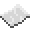# bit32.rshift

Bitwise right-shift. When called, shifts all bits in a number the specified amount to the right. For example, twelve is 1100 in binary. Shifting it two bits to the right makes it 3 (11). A right-shift is also equivalent to number / 2shift.ExampleShifting bits
Shifting ten (1010) 2 bits to the left. This should output 2, as 1010 becomes 10 (the last bit is dropped.)
Code
```<nowiki>
local ten = 10
print(bit32.rshift(ten, 2))
</nowiki>
```
Output 2

bit32.rshift
Function
Syntax
bit32.rshift(
• number : number
• shift : number
)

Returns number shifted
API bit32
Source Lua (source)